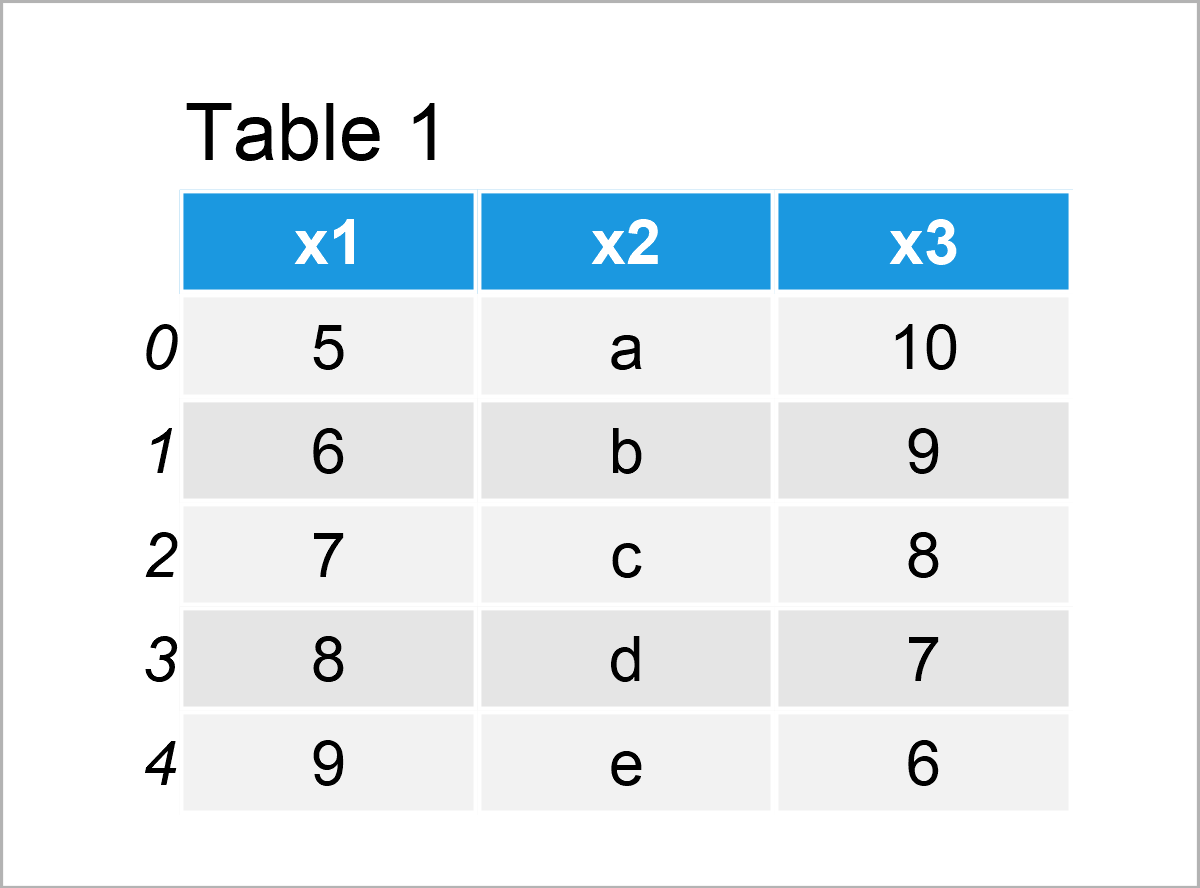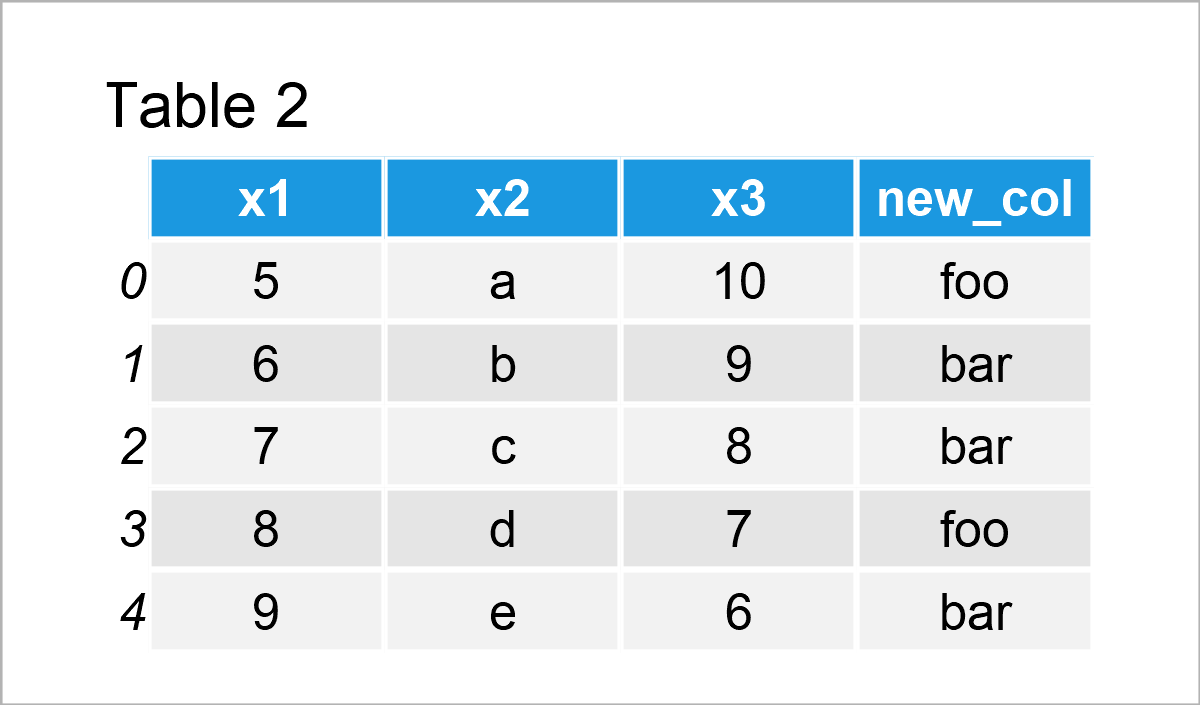# What is pandas in Python? (3 Examples)

This tutorial explains what the pandas library is and how to use it in the Python programming language.

Here’s move on to the definition…

## Definition of the pandas Library in Python

pandas is a software library that was created by Wes McKinney for the Python programming language.

The pandas library is mainly used for data manipulation, i.e. to edit, modify, and adjust certain components of a DataFrame object.

However, pandas is very flexible and can also be used for other tasks such as drawing data sets in plots, and storing time series values.

As other Python libraries, pandas is open source, i.e. freely available for usage, modification, and redistribution.

In the remaining part of this tutorial, I’ll show some example applications of the pandas library in practice.

So without too much talk, let’s dive into the example code!

## Example Data & Add-On Libraries

We first have to load the pandas library to Python:

`import pandas as pd                              # Import pandas library`

Next, we can use the pd.DataFrame function to create some example data:

```data = pd.DataFrame({"x1":range(5, 10),          # Create pandas DataFrame
"x2":["a", "b", "c", "d", "e"],
"x3":range(10, 5, - 1)})
print(data)                                      # Print pandas DataFrame```Table 1 shows that our example DataFrame is composed of five rows and three columns.

## Example 1: Add New Column to pandas DataFrame

This example illustrates how to append a new variable to a pandas DataFrame.

For this task, we first have to create a list object that contains the values of our new column:

```new_col = ["foo", "bar", "bar", "foo", "bar"]    # Create list
print(new_col)                                   # Print list
# ['foo', 'bar', 'bar', 'foo', 'bar']```

Next, we can apply the assign function to add our list as a new column to our pandas DataFrame:

```data_add = data.assign(new_col = new_col)        # Add new column
print(data_add)                                  # Print DataFrame with new column```As shown in Table 2, the previous code has managed to construct a new pandas DataFrame containing our input data plus our list object as a new variable.

## Example 2: Remove Row from pandas DataFrame

Example 2 shows how to drop certain rows from a pandas DataFrame.

To achieve this, we can use logical operators as illustrated below:

```data_drop = data[data.x2 != "c"]                 # Drop row using logical condition
print(data_drop)                                 # Print DataFrame without row```Table 3 shows the output of the previous Python syntax: We have excluded the third row from our data set.

## Example 3: Calculate Mean for pandas DataFrame Column

The pandas library can also be used to calculate certain descriptive statistics of the columns of a DataFrame.

In this specific example, we calculate the mean value of the variable x3:

```data_mean = data["x3"].mean()                    # Calculate average
print(data_mean)                                 # Print average
# 8.0```

The previous console output shows the mean value of our third column, i.e. 8.0.

## Video & Further Resources

I have recently released a video on my YouTube channel, which explains the contents of this tutorial. You can find the video below.

Furthermore, you may read the related articles on my website.

In summary: You have learned in this article how to apply the functions of the pandas library in the Python programming language. If you have any additional questions and/or comments, let me know in the comments section below.

Subscribe to the Statistics Globe Newsletter